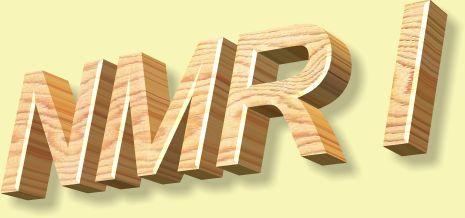1.* (1997 F 3) Perfluorocarbons are hydrocarbon derivatives in which every hydrogen atom has been substituted by a fluorine atome. Perfluorocarbons have many uses. For example, these liquids can dissolve large amounts of molecular oxygen and allow patients to breath oxygen through lungs full of liquid rather than gaseous air. Consider perfluorodecalin, whose structure was represented as shown below in a recent article (Chemistry in Britain, August 1997).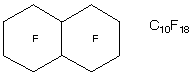A. Suppose you employed a simple gas chromatograph to anlyze perfluorodecalin. How many stereoisomers would you expect to find? Indicate the nature of these stereoisomers. B. 19F (100% natural abundance) has a nuclear spin of plus or minus 1/2 (like the proton). Explain the use of 19F NMR to analyze a sample of perfluorodecalin; explain the number of 19F resonances youwould expect to find in an unpurified sample (ignore spin coupling). Click here for solution to problem 1. 2.* (1997 2 1) Two 1H NMR spectra are shown, one of a substance X, C5H10O3 and another of its hydrolysis product Y, C2H6O. It is important to note that the spectrum of Y was performed with a trace of acid present. Furthermore, there is a small amount of TMS (tetramethylsilane) present in the spectrum of X but there was no TMS present in the spectrum of Y. The reaction converting X to Y is shown below as an unbalanced equation. A water soluble gas Z evolved during this reaction; a sample of Z showed no 1H NMR signal. The mass spectrum of Z revealed a parent ion at mass 44. The infrared spectrum of X showed a strong peak at about 1750 cm-1–a region associated with carbonyl groups in aldehydes, ketones and esters. No such peak was found in the infrared spectrum of Y.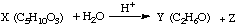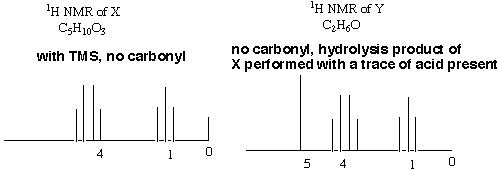On the basis of these data answer the following questions. A. How many sites of unsaturation in X, Y, and Z? B. What alkyl group is represented by the 1H NMR spectrum of X? C. Deduce the structure of X from its 1H NMR spectrum. On the 1H NMR spectrum of X write its structure and indicate which peaks are associated with each type of 1H NMR signal. D. Assume the alkyl group in X is retained in the hydrolysis product Y. On the 1H NMR spectrum of Y write a structural formula for Y and assign the peaks to each type of 1H NMR signal. E. How many 13C signals would you expect in the 13C NMR of X, if you ignore 1H-13C coupling? F. Write a structural formula for Z. G. What is the role of the trace of acid in the spectrum of Y? Explain in 2-3 sentences. Click here for solution to problem 2. 3.* (1996 F 3) Adenosine triphosphate, "ATP," is often called the energy currency of aerobic organisms. Oxidative phosphorylation results in energy stored by ATP; hydrolysis of ATP to ADP and phosphate releases energy. Below is a structural formula of ATP. Sketch the 31P NMR spectrum you might expect to be manifest by ATP; note that 31P (100% natural abundance) has nuclear spin 1/2. Do not ignore spin-coupling.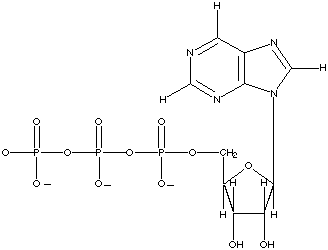Click here for solution to problem 3. 4.* (1996 2 5) The accompanying figure displays the 1H NMR spectrum of acetaldehyde, CH3CHO, measured at 60 MHz. By writing on the spectrum define the following quantities: A. chemical shift. B. spin coupling C. upfield (increasing magnetic field) D. Assign the peaks in this spectrum to their counterparts on the structure of acetaldehyde. E. In the space provided below sketch the proton NMR spectrum you expect for deuteroacetaldehyde: CH3CDO (recall that deuterium has a nuclear spin quantum number of 1).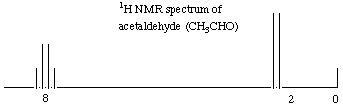Click here for solution to problem 4. 5.* (1996 2 6) A.How many different 13C NMR signals do you expect from the following ferrocene derivative (ignore spin coupling)?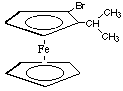B. On the structure below write arrows with numbers, etc. to each different carbon. Remember that the rings rotate rapidly with respect to one another. Warning! This question contains a hidden trick.Click here for solution to problem 5. 6.* (1995 2 1) Three 1H NMR spectra are displayed; X and Y represent substances having the molecular formula C8H12O4; Z has a formula C8H14O4. Reaction of either X or Y with H2 over a Pd metal catalyst forms Z. IR data shows that X, Y, and Z have one or more carbonyl-containing functional groups.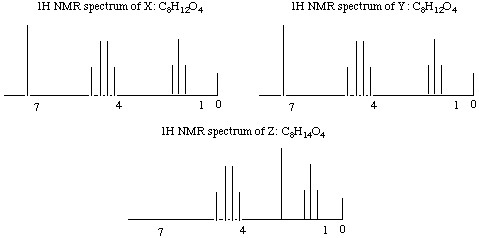A. Propose structures for X, Y, and Z by writing out structural formulas on each spectrum; make assignments of each proton type to the 1H NMR signals. Assign the signal at 0 shown on each spectrum. B. Do you have sufficient information to distinguish X from Y? C. Deuterium has a spin of 1; sketch the 1H NMR spectrum of D-H and compare this with the spectrum of H-H. Click here for solution to problem 6. 7.* (1994 F 2) The perfume industry can be traced back through many civilizations; for example, in the 5th centruy BC Herodotus included a chapter on perfumes in his "Histories." In modern times, some perfumes are made by synthetic organic chemists, while others are isolated from natural products. Consider a-isomethylionone, X, a component derived from violet flower oils. The structural formula of X is shown below in chichenwire notation.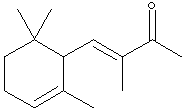A sample X (Xn) derived from natural flower oils is optically active; however, a sample of X (Xs) produced from a total synthesis does not rotate plane-polarized light even though this synthetic sample, Xs, exhibits both an infrared spectrum and 1H and 13C-NMR spectra identical to those of the sample Xn derived from natural sources. The natural and synthetic samples Xn and Xs have different fragrances. Catalytic hydrogenation of one mole of either Xn or Xs over a Pd catalyst took up to 2 moles of H2 (as indicated below). The product Ys from Xs was shown to be a mixture of 4 components. When the 4 components of product Ys were separated with a gas chromatograph, each component showed the same molecular formula (C14H26O) as determined by mass spectrometry. Xn, Xs, and the 4 components of Ys show a strong infrared peak in the region associated with a ketone (1690-1700 cm-1). On the basis of this information answer the following: A. How many sites of unsaturation are in X and Y, respectively? B. Write an asterisk beside each chiral center in the structural formula shown above for X. C. Identify the nature of the apparent stereoisomers: Xn and Xs. D. Write the structural formula for Y in chickenwire notation. E. Place asterisks beside each chiral center on your structure for Y. F. What is the nature of the four stereoisomeric components represented by Y? A simple term will do. G. Using the terms R and S, explain why Yn would exist as 4 stereoisomers. H. Now consider hydrogenation of Xs to form Ys. How many components would you expect to separate from the product Ys? Would any of these exhibit optical activity? Explain in two sentences. I. How many methyl signals would you expect to see in the 13C NMR spectrum of Xs (ignore spin coupling). J. Which of the methyl signals would resonate at lowest field (i.e. have the largest chemical shift)? K. Could you separate Ys into even more components with a chromatography column; if so indicate a crucial property of such a column? L. Do you expect the 4 components Ys to have different fragrances than Xn? Explain. Click here for solution to problem 7.